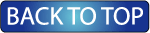Oceanographic Observations, Hudson Shelf Valley, U.S. Geological Survey Open-File Report 02-217

## Statistics of Current andTemperature Observations

Statistics were calculated for the hour-averaged and low-pass filtered measurements, for the entire data set and by month.

### Current Statistics for Entire Deployment

#### Statistics by Month

The statistics table includes the number of data points used to calculate statistics for the given time period (number). For hour-averaged data, the number is the number of hours of data available in each month. If the number of data points is not equal to 672, 720, or 744 hours for months with 28, 30 or 31 days respectively, then the data is not complete for that month. For the low-pass filtered data that is subsampled every 6 hours, if the number of data points is not equal to 112, 120, or 124 for months with 28, 30 or 31 days respectively, then the data set is not complete for that particular month. Gaps in the data set are due to instrument malfunctions.

*Note, "No Data" means no temperature measurements were made at that depth at that station.

### Hour-Averaged Data

 Station Surface Mid-Depth (1) Mid-Depth (2) Bottom A No Data No Data B C D No Data No Data E No Data No Data F No Data No Data No Data All Stations combined plots combined plots combined plots combined plots

### Low-Passed Data

 Station Surface Mid-Depth (1) Mid-Depth (2) Bottom A No Data No Data B C D No Data No Data E No Data No Data F No Data No Data No Data All Stations combined plots combined plots combined plots combined plots